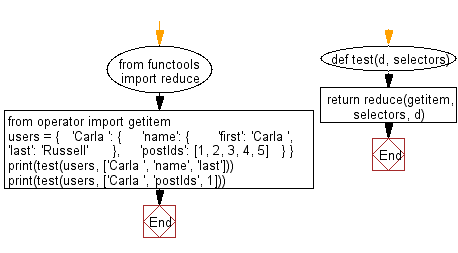﻿ Python: Retrieve the value of the nested key indicated by the given selector list from a dictionary or list - w3resource# Python: Retrieve the value of the nested key indicated by the given selector list from a dictionary or list

## Python dictionary: Exercise-71 with Solution

Write a Python program to retrieve the value of the nested key indicated by the given selector list from a dictionary or list.

Sample Solution:

Python Code:

``````from functools import reduce
from operator import getitem
def test(d, selectors):
return reduce(getitem, selectors, d)
users = {
'Carla ': {
'name': {
'first': 'Carla ',
'last': 'Russell'
},
'postIds': [1, 2, 3, 4, 5]
}
}
print(test(users, ['Carla ', 'name', 'last']))
print(test(users, ['Carla ', 'postIds', 1]))
```
```

Sample Output:

```Russell
2
```

Flowchart:## Visualize Python code execution:

The following tool visualize what the computer is doing step-by-step as it executes the said program:

Python Code Editor:

Have another way to solve this solution? Contribute your code (and comments) through Disqus.

What is the difficulty level of this exercise?

Test your Programming skills with w3resource's quiz.

﻿

## Python: Tips of the Day

Decapitalizes the first letter of a string:

Example:

```def tips_decapitalize(s, upper_rest=False):
return s[:1].lower() + (s[1:].upper() if upper_rest else s[1:])
print(tips_decapitalize('PythonTips'))
print(tips_decapitalize('PythonTips', True))
```

Output:

```pythonTips
pYTHONTIPS
```